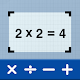Home » Education » Math Scanner - Math Solutions# Math Scanner - Math Solutions

Pic Frame Photo Collage Maker \u0026 Picture Editor

(68205)
Take a photo to solve math problem & get a math solution & equation calculation

## The description of Math Scanner - Math Solutions

Math Scanner by Photo - Solve My Math Problem Maths will give you the answer to any math question by simply taking a photo of it. With millions of users and many problems solved, math way is the world's smartest math calculator app. With a smart calculator, you just need to enter the formula and the equation calculator will give you answers automatically. Simply point your camera toward a problem and photo calculator will magically show the result with detailed step-by-step instructions. The calculator will give you the answer to any math question by simply taking a photo of it. The calculator is a great way to check your homework, study and learn math. Supports arithmetic, integers, fractions, decimal numbers, roots, algebraic expressions, linear equations/inequalities, quadratic equations/inequalities, absolute equations/inequalities, systems of equations, logarithms, trigonometry, exponential and logarithmic functions, derivatives and integrals, graphs and many more.

Features of Math Scanner - Solve My Math Problem:

# Calculate math problems with the camera.
# Math scanner with no internet required.
# Step by step solutions for easy learning.
# Smart calculator with graphing and tables.
# Can solve simple to the advanced math question.
# Addition, subtraction, multiplication, division, algebra, percentage, EMI, interest, etc can be solved.
# Click a photo of any mathematical problem & solve that problem for you.

Math scanner - solve my math problem you can scan the problems and get quick and accurate math answers. This solve math by camera app will assist you with all the problems when you are doing homework. Photo calculator solver is a valuable tool for a parent helping with his kid 's math homework and attempting to remember middle-school algebra, photo calculator, take a photo to solve math. Our problem solver is designed to help you with your math homework. In addition to homework answers, we also show you how to get there step-by-step. Let me help you with your apps math homework today.

Solve math by camera Calculator is a useful and easy to use a calculator with a basic calculator, scientific calculator and also an equation solver and a great maths homework solver which can solve maths problems more efficiently, quickly and accurately by the camera. This is cymath answers for math help with math solver online to photo math solver. How to solve math problems is photomath apps to solve my problem solving app. That is answer my problems with math solution app or math problems with answers. A photomath android or math answer app to solve any math equation solver.

Math scanner - solve my math problem the photomath camera calculator is solver camera about math solving website or mathematics solver app for homework answers app. You can mathematics resolver and calculator for mathway offline. That is my script calculator to scan problems by photo or word problem solver app. This is calculator vault download algebra calculator photo with a financial calculator app. Will give you currency converter or currency calculator for money converter. This is BMI calculator with maths questions answering app to google maths solver in math question solver.

Camera calculator solver can simply snap photo calculator and solver instantly delivers the photo calculator answers for math solution while also providing step by step solution. You can mathematics apps and math homework answers to solve the sum app. A photomath with solution offline with photo math trigonometry calculator for math quiz games. For math education and math word problem solver with math photo scanner to math tutor app. Any other problems such as calculus, equation systems, complex math, graphing, table of values, and more solutions.

Download and give us a review of Math Scanner - Solve My Math Problem.
Category: Free Education App
Publish Date: September 21, 2022